# Fraction on the Number Line

Fractions expand our number system beyond whole numbers and integers. They help us denote any decimal number with higher precision. Representation of Fractions on the number line is important to understand how fractions represent part of a whole.

We all know that a fraction is a number which is not a whole number,  (such as 1/2, 5/7 etc). Let us see how fractional numbers are represented on the number line.

## Representing Fractions on the Number Line

We already know how to represent whole numbers on the number line. Let us consider the image shown, here, the digits 0 and 1 are represented on the number line.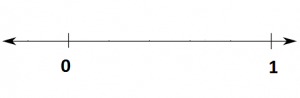If we divide the number line into two equal parts, as shown in the figure below, what value does that mid-point hold? Half of what the graduation between 0 and 1 holds.

So, the point represents (1-0)/2 = ½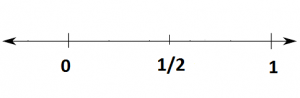Thus we saw, to represent a fraction on the number line, we need to divide the line segment between two whole numbers into n equal parts where n represents the denominator of the fraction. For example, if we have to represent the fraction 1/5 on the number line, hence, we need to divide the line segment between 0 and 1 into five equal parts, where the first point as shown in the figure represents the fraction 1/5, the second represents 2/5 and so on.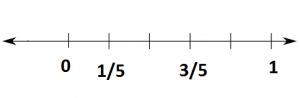We must note that, 0/5 = 0 here and 5/5 = 1.

Here you have seen the representation of proper fraction on the number line. To represent improper fractions, you first convert them into mixed fractions. The same procedure, as shown above, is used with the whole number as the starting point.

### Absolute Value and the Number Line

The below number line represents the integers that include from -6 to +6. Here, the positive integers go to the right of 0 such as 1, 2, 3, and so on whereas, the negative integers go to the left of 0 such as -1, -2, -3, and so on.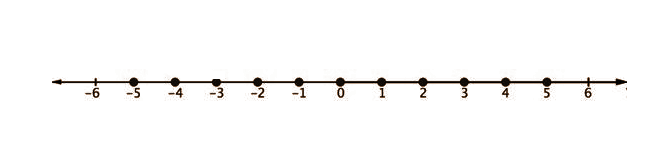The distance between 0 and the number place on the number line is called the number’s absolute value. The absolute value of the number can be denoted using short vertical lines on either side of the number. For example, the absolute value of -6 is written as |-6|. It is noted that the distance is always a positive value or 0.

### Fractions on the Number Line Example

Let us understand the representation with the help of a simple example.

Example:

Represent 43/5 on the number line.

Solution:

First, convert 43/5 into the mixed fraction.

$$\begin{array}{l}\frac{43}{5} = 8\frac{3}{5}\end{array}$$

Now, we start from 8 and divide the section between 8 and 9 into 5 equal parts (5 being the denominator). The third point, as shown in the figure, represents our required fraction.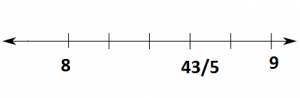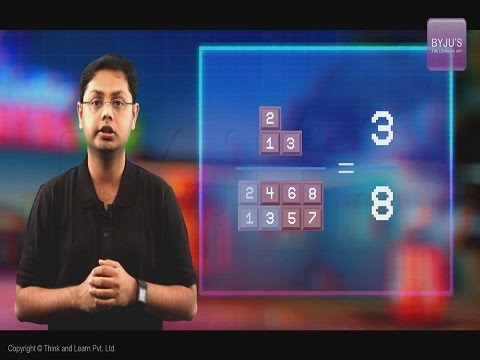To solve more problems on the topic, download BYJU’S – The Learning App from Google Play Store and watch interactive videos. Also, take free tests to practice for exams.